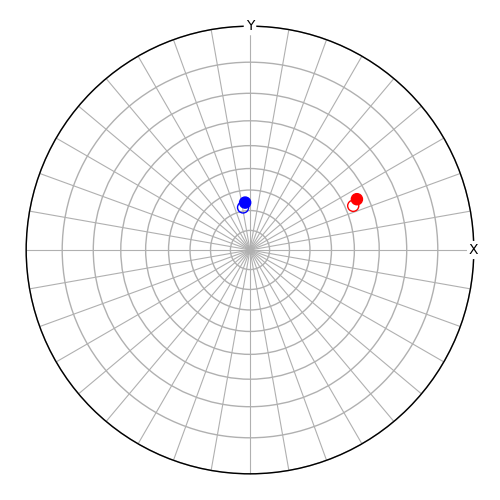# Orientation from aligning directions#

This example demonstrates how to use `from_align_vectors()` to estimate an orientation from two sets of aligned vectors, one set in the sample reference reference frame, the other in the crystal reference frame.Out:

```Vector angle deviation [deg]:  1.1551484899021243
Error distance:  0.02218779280928904
```

```from diffpy.structure import Lattice, Structure
import numpy as np

from orix.crystal_map import Phase
from orix.quaternion import Orientation
from orix.vector import Miller, Vector3d

# Specify a crystal structure and symmetry
phase = Phase(
point_group="6/mmm",
structure=Structure(lattice=Lattice(1, 1, 2, 90, 90, 120)),
)

# Define a reference orientation (goal)
o_ref = Orientation.from_axes_angles([1, 1, 1], 45, phase.point_group, degrees=True)

# Specify two crystal directions (any will do)
v_c = Miller(uvw=[[2, 1, 1], [1, 3, 1]], phase=phase)

# Find out where these directions in the reference orientation (crystal)
# point in the sample reference frame
v_r = Vector3d(~o_ref * v_c)

# Plot the reference orientation sample directions as empty circles
fig = v_r.scatter(
ec=["r", "b"],
s=100,
fc="none",
grid=True,
axes_labels=["X", "Y"],
return_figure=True,
)
fig.tight_layout()

# Add some randomness to the sample directions (0 error magnitude gives
# exact result)
err_magnitude = 0.1
v_err = Vector3d(np.random.normal(0, err_magnitude, 3))
v_r_err = v_r + v_err
angle_err = v_r_err.angle_with(v_r, degrees=True).mean()
print("Vector angle deviation [deg]: ", angle_err)

# Obtain the orientation which aligns the crystal directions with the
# sample directions
o_new_r2c, err = Orientation.from_align_vectors(v_c, v_r_err, return_rmsd=True)
print("Error distance: ", err)

# Plot the crystal directions in the new orientation
v_r2 = Vector3d(~o_new_r2c * v_c)
v_r2.scatter(c=["r", "b"], figure=fig)
```

Total running time of the script: ( 0 minutes 0.407 seconds)

Estimated memory usage: 9 MB

Gallery generated by Sphinx-Gallery